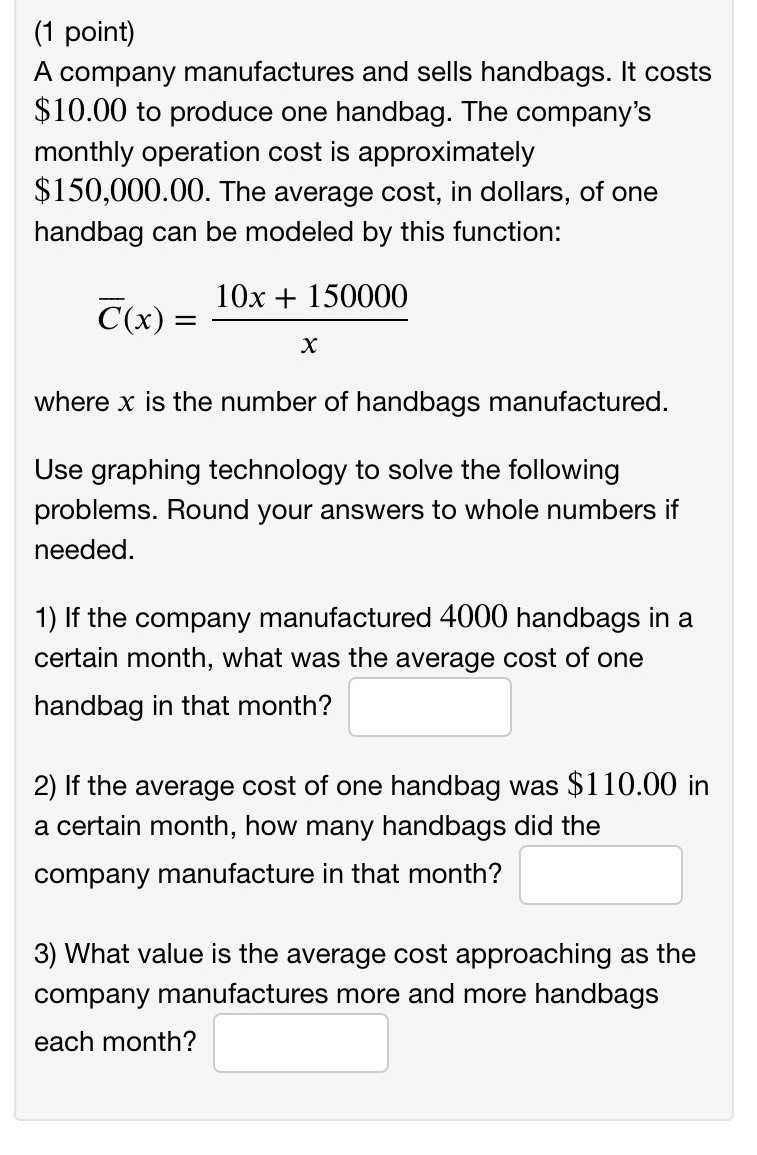### Still have math questions?

Algebra
QuestionA company manufactures and sells handbags. It costs $$\ 10.00$$ to produce one handbag. The company's monthly operation cost is approximately $$\ 150,000.00$$ . The average cost, in dollars, of one handbag can be modeled by this function:

$$\overline { C } ( x ) = \frac { 10 x + 150000 } { x }$$

where $$x$$ is the number of handbags manufactured.

Use graphing technology to solve the following problems. Round your answers to whole numbers if needed.

1) If the company manufactured $$4000$$ handbags in a certain month, what was the average cost of one handbag in that month?

2) If the average cost of one handbag was $$\ 110.00$$ in a certain month, how many handbags did the company manufacture in that month?

3) What value is the average cost approaching as the company manufactures more and more handbags each month?